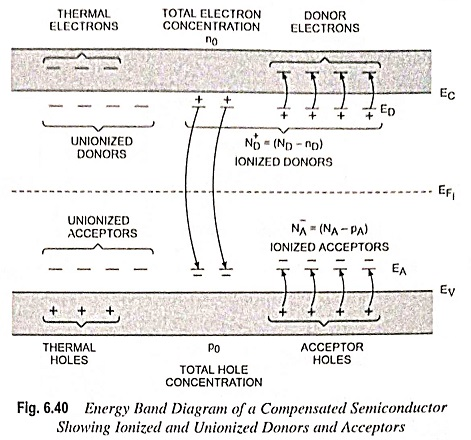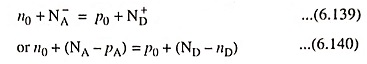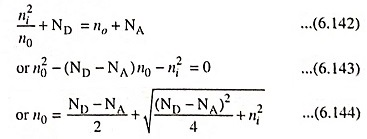## Charge Neutrality Equation in Semiconductor:

The semiconductor crystal is electrically neutral under thermal equilibrium conditions. The electrons are distributed among the different energy states, producing both negative and positive charges but the net charge density is zero. This charge neutrality condition is used for determination of the thermal-equilibrium electron and the hole concentrations as a function of impurity doping concentration.

### Compensated Semiconductors:

A semiconductor containing both donor and acceptor impurity atoms in the same region is called a compensated semiconductor, It is formed by diffusing donor impurities into a P-type material (NA > ND) or by diffusing acceptor impurities into an N-type material (ND > NA). If ND = NA, we have a completely compensated conductor. Compensated conductors are produced quite naturally during device fabrication.

Energy hand diagram of a compensated semiconductor is shown in Fig. 6.40. The figure depicts how the electrons and holes can be distributed among the various states.The charge density of negative and positive charges can be equated for charge neutrality condition and thus we havewhere p0 and n0 are the thermal-equilibrium concentrations of holes and electrons in the valence and conduction bands respectively. The parameter pA is the concentration of holes in the acceptor energy states, so NA = NA – pA is the concentration of negatively charged acceptor states. Similarly, nD is the concentration of electrons in the donor energy states, so N+= ND – nD is the concentration of positively charged donor states. For complete ionization nD and PA are both zero so that Eq. (6.140) becomesIf is expressed as n2i/n0, thenThe positive sign in the quadratic equation is required to be used, since, in the limit of an intrinsic semiconductor when NA = ND = 0, the electron concentration must be a positive quantity, or n0 = ni.

Above Eq. (6.144) is used to determine the electron concentration in an N-type semiconductor or when ND > NA. This equation is also valid for NA = 0.

Scroll to Top